# Video Compression Basics - MPEG2

31 Oct 2009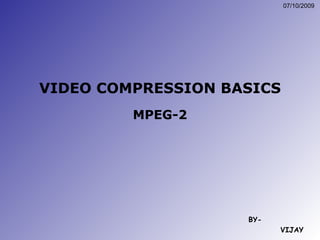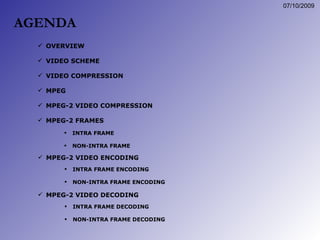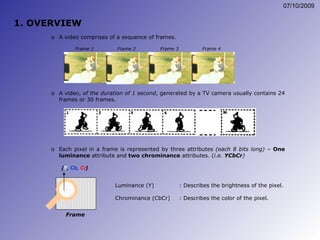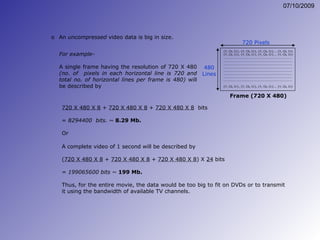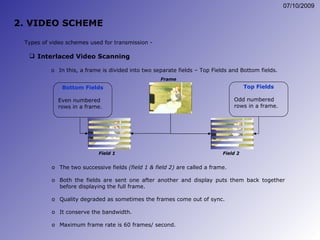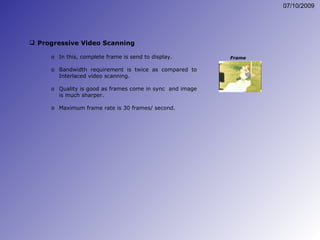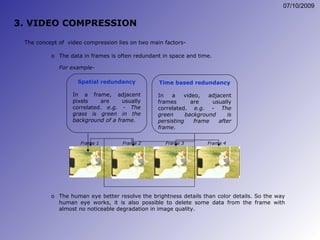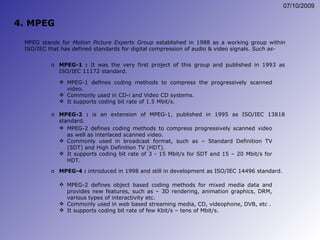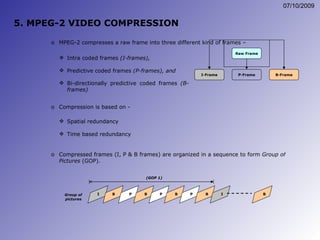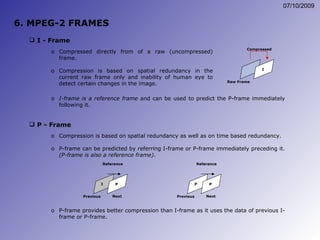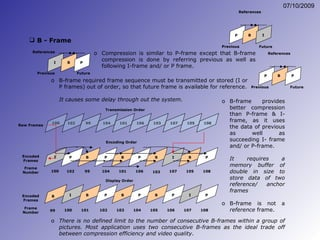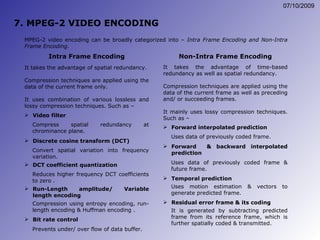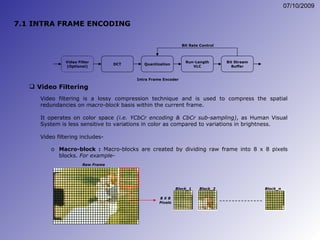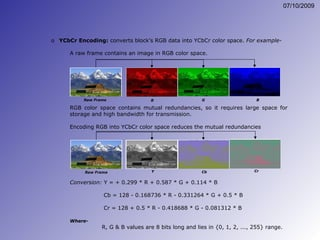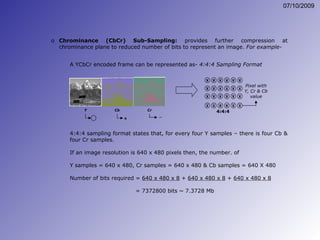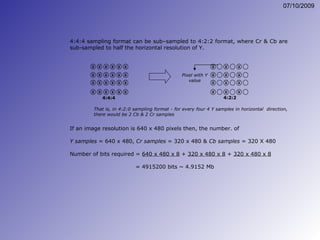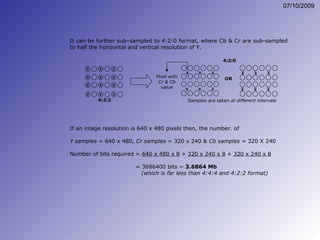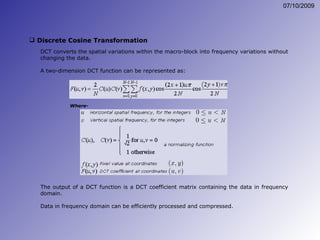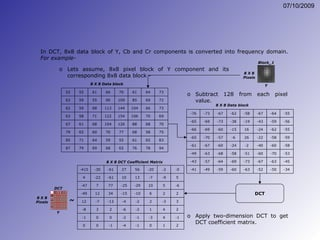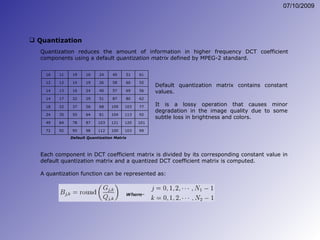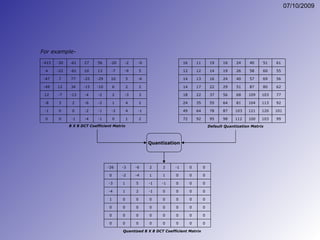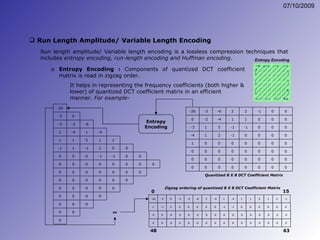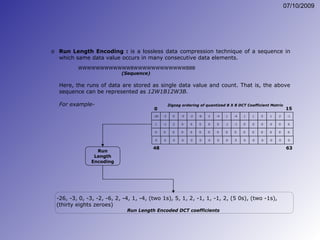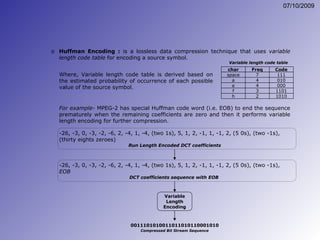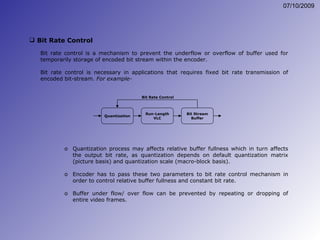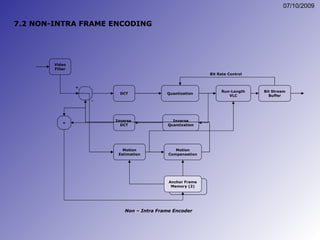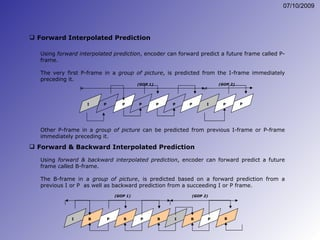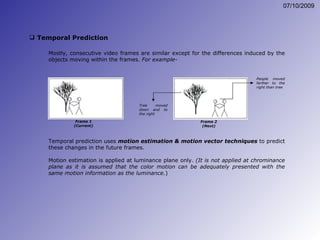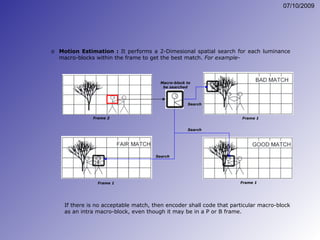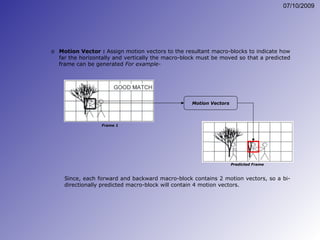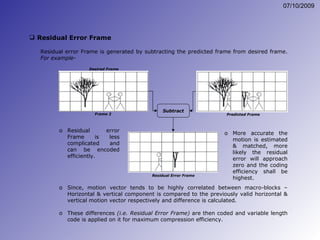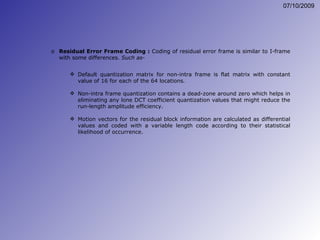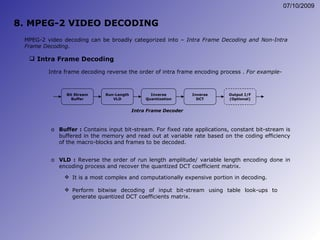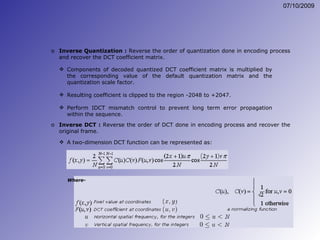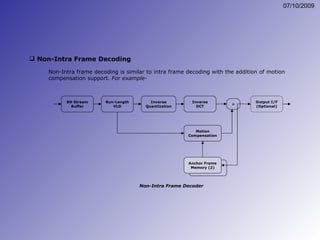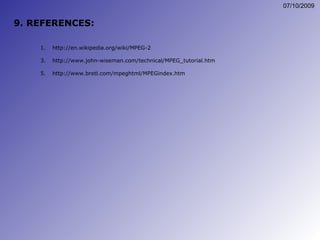1 sur 37

### Video Compression Basics - MPEG2

• 1. VIDEO COMPRESSION BASICS MPEG-2 BY- VIJAY
• 2.
• 3.
• 4.
• 5.
• 6.
• 7.
• 8.
• 9.
• 10.
• 11.
• 12.
• 13.
• 14.
• 15.
• 16. 4:4:4 sampling format can be sub–sampled to 4:2:2 format, where Cr & Cb are sub-sampled to half the horizontal resolution of Y. That is, in 4:2:0 sampling format - for every four 4 Y samples in horizontal direction, there would be 2 Cb & 2 Cr samples If an image resolution is 640 x 480 pixels then, the number. of Y samples = 640 x 480, Cr samples = 320 x 480 & Cb samples = 320 X 480 Number of bits required = 640 x 480 x 8 + 320 x 480 x 8 + 320 x 480 x 8 = 4915200 bits ~ 4.9152 Mb x x x x x x x x x x x x x x x x x x x x x x x x 4:4:4 x x x x x x x x x x x x 4:2:2 Pixel with Y value
• 17. It can be further sub–sampled to 4:2:0 format, where Cb & Cr are sub-sampled to half the horizontal and vertical resolution of Y. If an image resolution is 640 x 480 pixels then, the number. of Y samples = 640 x 480, Cr samples = 320 x 240 & Cb samples = 320 X 240 Number of bits required = 640 x 480 x 8 + 320 x 240 x 8 + 320 x 240 x 8 = 3686400 bits ~ 3.6864 Mb (which is far less than 4:4:4 and 4:2:2 format) 4:2:0 OR Pixel with Cr & Cb value Samples are taken at different intervals x x x x x x x x x x x x x x x x x x x x x x x x 4:2:2
• 18.
• 19.
• 20.
• 21. Quantization For example- -26 -3 -6 2 2 -1 0 0 0 -2 -4 1 1 0 0 0 -3 1 5 -1 -1 0 0 0 -4 1 2 -1 0 0 0 0 1 0 Quantized 8 X 8 DCT Coefficient Matrix 0 0 0 0 0 0 0 0 0 0 0 0 0 0 0 0 0 0 0 0 0 0 0 0 0 0 0 0 0 0 -415 -30 -61 27 56 -20 -2 -0 4 -22 -61 10 13 -7 -9 5 -47 7 77 -25 -29 10 5 -6 -49 12 34 -15 -10 6 2 2 12 -7 -13 -4 -2 2 -3 3 -8 3 2 -6 -2 1 4 2 -1 0 0 -2 -1 -3 4 -1 0 0 -1 -4 -1 0 1 2 8 X 8 DCT Coefficient Matrix 16 11 19 16 24 40 51 61 12 12 14 19 26 58 60 55 14 13 16 24 40 57 69 56 14 17 22 29 51 87 80 62 18 22 37 56 68 109 103 77 24 35 55 64 81 104 113 92 49 64 78 87 103 121 120 101 72 92 95 98 112 100 103 99 Default Quantization Matrix
• 22.
• 23.
• 24.
• 25.
• 26. 7.2 NON-INTRA FRAME ENCODING Video Filter DCT Quantization Run-Length VLC Bit Stream Buffer Inverse Quantization Inverse DCT Motion Estimation Motion Compensation Anchor Frame Memory (2) + + - Bit Rate Control Non – Intra Frame Encoder
• 27.
• 28.
• 29.
• 30.
• 31.
• 32.
• 33.
• 34.
• 35.
• 36.
• 37. THANK YOU!